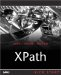# Copying Nodes

It's often useful to be able to simply copy nodes from the input document to the output document without making any changes, and we'll take a look at how that works here, with examples. There are two elements that you can use to copy nodes in XSLT <xsl:copy> and <xsl:copy-of> .

#### Using the <xsl:copy> Element

The <xsl:copy> element lets you copy a node from the source tree to the output tree. Note that this is a shallow copy, however, which means that it does not copy any of the node's descendants. No attributes of elements are copied either.

<XSL:COPY> AND ROOT NODES

Using <xsl:copy> actually does nothing when used on the root node, because the root of the output document is created automatically.

This element can contain a template body, which is used only when the node to copy is a root node or an element.

You can see an example in ch05_06.xsl in Listing 5.5all this example does is to copy all elements from the source document to the result document, using <xsl:copy> .

##### Listing 5.5 An XSLT Stylesheet That Copies Elements ( ch05_06.xsl )
` <?xml version="1.0" encoding="UTF-8"?> <xsl:stylesheet version="1.1"  xmlns:xsl="http://www.w3.org/1999/XSL/Transform">     <xsl:output method="xml"/>     <xsl:template match="*">  <xsl:copy>   <xsl:apply-templates/>   </xsl:copy>  </xsl:template> </xsl:stylesheet> `

However, note that <xsl:copy> does not copy attributes, so here's the result when we use this stylesheet on ch05_01.xml :

` `
` <?xml version="1.0" encoding="UTF-8"?> <planets>     <planet>         <name>Mercury</name>         <mass>.0553</mass>         <day>58.65</day>         <radius>1516</radius>         <density>.983</density>         <distance>43.4</distance>     </planet>     <planet>         <name>Venus</name>         <mass>.815</mass>         <day>116.75</day>         <radius>3716</radius>         <density>.943</density>         <distance>66.8</distance>     </planet>     <planet>         <name>Earth</name>         <mass>1</mass>         <day>1</day>         <radius>2107</radius>         <density>1</density>         <distance>128.4</distance>     </planet> </planets> `

You can also copy attributes if you find a way to apply <xsl:copy> to each of an element's attributes. That can be done, for example, with <xsl:for-each> , as you see in ch05_07.xsl in Listing 5.6.

##### Listing 5.6 An XSLT Stylesheet That Copies Elements and Attributes ( ch05_07.xsl )
` <?xml version="1.0" encoding="UTF-8"?> <xsl:stylesheet version="1.1"  xmlns:xsl="http://www.w3.org/1999/XSL/Transform">     <xsl:output method="xml"/>     <xsl:template match="*">  <xsl:copy>   <xsl:for-each select="@*">   <xsl:copy/>   </xsl:for-each>   <xsl:apply-templates/>   </xsl:copy>  </xsl:template> </xsl:stylesheet> `

Here's the resultnote that this time, the attributes are intact:

` `
` <?xml version="1.0" encoding="UTF-8"?> <planets>     <planet>         <name>Mercury</name>         <mass units="(Earth = 1)">.0553</mass>         <day units="days">58.65</day>         <radius units="miles">1516</radius>         <density units="(Earth = 1)">.983</density>         <distance units="million miles">43.4</distance>     </planet>     <planet>         <name>Venus</name>         <mass units="(Earth = 1)">.815</mass>         <day units="days">116.75</day>         <radius units="miles">3716</radius>         <density units="(Earth = 1)">.943</density>         <distance units="million miles">66.8</distance>     </planet>     <planet>         <name>Earth</name>         <mass units="(Earth = 1)">1</mass>         <day units="days">1</day>         <radius units="miles">2107</radius>         <density units="(Earth = 1)">1</density>         <distance units="million miles">128.4</distance>     </planet> </planets> `

However, there's an easier way of making sure that you copy all the children, attributes, and other descendants of nodesyou can use <xsl:copy-of> instead of <xsl:copy> .

#### The <xsl:copy-of> Element

The <xsl:copy-of> element lets you make a deep copy of nodes, which means that the node and all its attributes and descendants are copied. This element has one attribute, select , which is mandatory and specifies the node or node-set you want copied. This element is empty, and takes no content.

Here's an example showing how this works; in this case, we'll just replace the <xsl:for-each> element in the preceding example with an <xsl:copy-of> element that specifically selects all attributes of the context element to copy. You can see what this looks like in ch05_08.xsl in Listing 5.7.

##### Listing 5.7 An XSLT Stylesheet That Copies Elements and Attributes ( ch05_08.xsl )
` <?xml version="1.0" encoding="UTF-8"?> <xsl:stylesheet version="1.1"  xmlns:xsl="http://www.w3.org/1999/XSL/Transform">     <xsl:output method="xml"/>  <xsl:template match="*">   <xsl:copy>   <xsl:copy-of select="@*"/>   <xsl:apply-templates/>   </xsl:copy>   </xsl:template>  </xsl:stylesheet> `

This works as the example in the previous section did, copying all elements and attributes. In fact, we don't need to modify the previous example at allwe can simply use <xsl:copy-of> to copy the entire document by matching the root node and copying all descendants of that node like this:

` `
` <?xml version="1.0" encoding="UTF-8"?> <xsl:stylesheet version="1.1"  xmlns:xsl="http://www.w3.org/1999/XSL/Transform">     <xsl:output method="xml"/>  <xsl:template match="/">   <xsl:copy-of select="*"/>   </xsl:template>  </xsl:stylesheet> `

You can also use <xsl:copy-of> to copy particular nodes and their descendants instead of matching the wildcard "*". For example, this rule copies all <density> elements and their descendants:

` `
` <xsl:template match="density">     <xsl:copy-of select="."/> </xsl:template> `

You could even be tricky and replace the <density> element with a <mass> element like this when you perform the copy:

` `
` <xsl:template match="density">    <xsl:copy-of select="mass"/> </xsl:template> `XPath Kick Start: Navigating XML with XPath 1.0 and 2.0
ISBN: 0672324113
EAN: 2147483647
Year: 2002
Pages: 131

Similar book on Amazon